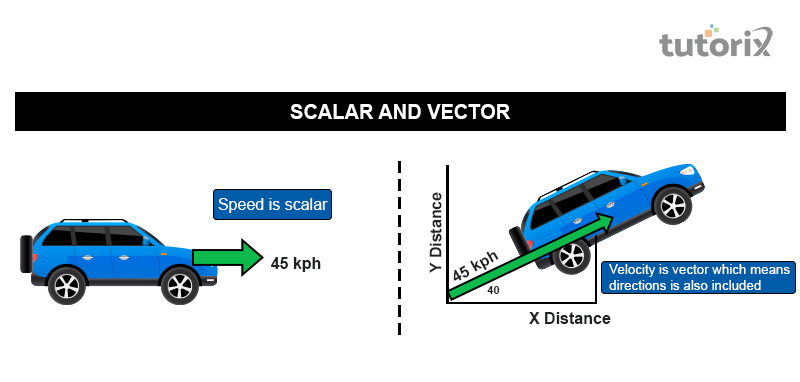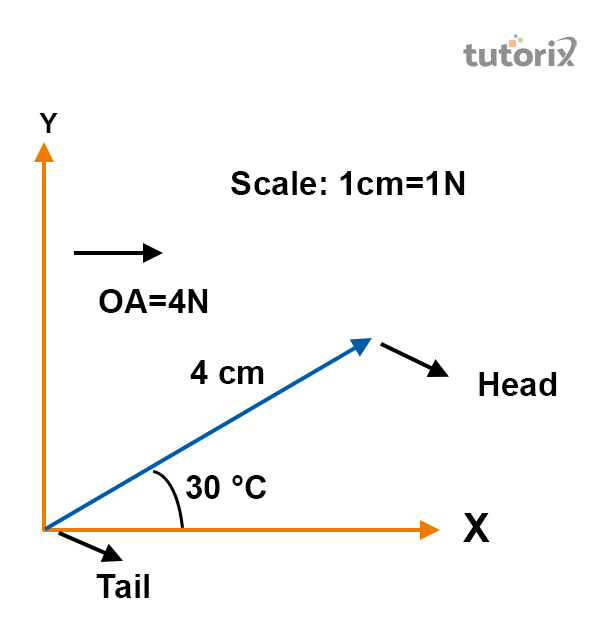# Difference Between Scalar and Vector

## Introduction

A vector and a scalar quantity can be explained with the help of algebraic principles. A scalar quantity represents a one-dimensional value whereas the vector quantity represents two and more than two-dimensional values. The motion of an object can also be represented with the help of a vector and a scalar quantity.

## What is Scalar?

An important mathematical expression of physics is a scalar quantity. This field of physics is associated with measurement. Meter and degree are the two most important units of measurement that are used in presenting the value of a scalar unit (Sirunyan et al. 2018). A numerical value is used to present the value of a scalar quantity. Size and magnitude are related terms of a scalar quantity

### Examples of a scalar quantity

• Charge density

• Mass

• Temperature

• Gravitational force

• Electric charge

• Volume

• Time

## What is a vector?

In a vector quantity, directions and magnitude act as two independent qualities. Magnitude is used to present the size of a vector quantity. Direction is used to denote the direction of any object’s travelling direction. The “triangle law of addition” is associated with vector quantity (Shadmi & Weiss, 2019). Because of having direction as an attribute of a vector quantity, two and even three-dimensional quantity is available for a vector quantity.Figure 1: Difference between Vector and Scalar quantity

Based on the above-presented figure, speed is the scalar quantity of the car. The velocity is denoted as the vector quantity of the car. The calculated velocity of 45 Kph is the value of velocity which is a vector quantity

• Force

• Electric field

• Acceleration

• Velocity

• Polarization

• Displacement

• Linear momentum

### Vector notation

Rectangular notation − In an important and significant rectangular coordinating system, the vector is located. Geometric representation is presented as followsFigure 2: Representation of a vector

As presented in the above figure, a vector quantity is situated on the X and Y-axis. The angle, created within vector quantity and X-axis is 300. The vector quantity is calculated as 4 cm.

At certain times, by following some basic rule, vectors can be added in a geometric manner. The addition process is dependent on some factors. Each behavioural character of a vector is determined based on individual characteristics (Alonso-Álvarez, Hugle & Jaeckel, 2020). The outcome of different vectors that acts on a particular body is regarded as a vector addition process. The property of communication is an important part of vector addition. The equation of property communication is as follows −

$$\mathrm{\overrightarrow{A} + \overrightarrow{B} = \overrightarrow{B} + \overrightarrow{A}}$$

### Vector subtraction

Subtraction of two vectors can be done by putting both vectors’ feet together. A resultant vector is to be drawn after that for subtracting vectors

Difference between Scalar and vector

Different parameters Vector Scalar
Meaning A vector quantity possesses both direction and magnitude. This quantity possesses no direction, only magnitude.
Change Changes are found in vector quantities when each of the magnitude and direction or even both of these change. Changes only in magnitude are responsible for the change in scalar quantity.
Quantities The result of a mathematical operation within two and even more vector quantities can provide results in both scalar and vector. Mathematical operations within two and more than two scalar quantities result in providing only scalar quantities. In the case of an operation between a scalar and a vector quantity, the result is provided as a vector quantity.
Operation The result of a mathematical operation within two and even more vector quantities can provide results in both scalar and vector. Mathematical operations within two and more than two scalar quantities result in providing only scalar quantities. In the case of an operation between a scalar and a vector quantity, the result is provided as a vector quantity.
Resolution A vector quantity can be easily resolved in any different direction with the help of sine, or cosine based on the adjacent angle (Baumann et al. 2020). Being of the same value of direction, a scalar quantity cannot be resolved.
Expression Boldface letters, such as V is used to present the value of velocity. Putting an arrowhead is another important term used in expressing vector values. Scalar values are expressed with simple alphabets such as V for velocity.
Division A vector quantity cannot be divided by any other vector. A scalar can be easily divided by another scalar quantity.
Measurement The vector value is measured in a complex manner. The measurement of a scalar value is simpler than a scalar value.

### Similarities between Scalar and vector

• Finite magnitude is found in both vector and scalar quantity.

• Both the vector and a scalar quantity are measurable with suitable instruments

• Both of these quantities possess specific units and dimensions (Zou & Myung, 2019).

• Scalar and vector quantities are expressed with certain physics quantities.

## Conclusion

At the time of providing a description of any physical quantity, magnitude and direction are needed. A vector quantity is relatable at this point. Some standard rules of algebra such as multiplication, addition, and subtraction are used to calculate the value of scalar quantities. Algebraic principles are followed by vector quantities.

### FAQs

Q1. What is the reason behind an electric current, not being a vector?

In the opposite direction of an electron flow, the electric current flows. A current possesses both, direction and magnitude. As a result, the electric current is a scalar

Q2. What is the magnitude value of a unit vector?

In a unit vector, no dimension is available. A magnitude of one is found in a unit vector. Unity is also regarded as the “magnitude of a united vector”.

Q3. What is the possibility of adding two vectors?

There is no possibility of adding two different vectors. In combining, vectors with the same quality, nature, and type are required. As an example, one force vector cannot be combined with a velocity vector, whereas two force vectors can be combined

Q4. What is mandatory while defining a vector quantity?

Definition of a vector quantity requires specification of direction. Velocity, weight, displacement, and force are some examples of a vector quantity.

Updated on: 13-Oct-2022

1K+ Views